These functions extend autoplot.sp() and autoplot.elev() and return not a single plot but a list of plots. They are particularly useful if you want to print a .pdf file with one plot per page. They automatically plot the variables sp and elev of a ForestGEO-like dataset of class 'sp' or 'sp_elev'.

• Create a 'sp' object with:

    object <- sp(DATA-WITH-VARIABLE-sp)

• Create a 'sp_elev' object with:

    object <- sp_elev(DATA-WITH-VARIABLE-sp, DATA-WITH-VARIABLE-elev)

See sections Usage and Examples.

# S3 method for sp_elev
autoplot_by_species(
object,
species = "all",
fill = "black",
shape = 21,
point_size = 3,
contour_size = 0.5,
low = "blue",
high = "red",
hide_color_legend = FALSE,
bins = NULL,
label_size = 3,
label_color = "grey",
xyjust = 1,
fontface = "italic",
xlim = NULL,
ylim = NULL,
custom_theme = NULL,
...
)

# S3 method for sp
autoplot_by_species(
object,
species = "all",
fill = "black",
shape = 21,
point_size = 3,
hide_color_legend = FALSE,
xlim = NULL,
ylim = NULL,
custom_theme = NULL,
...
)

## Arguments

object

An object created with sp() or sp_elev().

species

A character vector giving values in the column sp. The output will be a list with as many plots as elements in this vector. The string "all" (default) plots all unique values of sp.

fill

Character; either a color or "sp", which maps each species to a different color.

shape

A number giving point shape (as in graphics::points()). Passed to ggplot2::geom_point().

point_size

A number giving point size. Passed to ggplot2::geom_point().

contour_size

A number giving the size of the contour of elevation lines. Passed to ggplot2::stat_contour() (see ggplot2::geom_contour()).

low, high

A string giving a color of the elevation lines representing low and high elevation.

hide_color_legend

Logical; TRUE hides the color legend.

bins

A number giving the number of elevation lines to plot.

Logical; FALSE hides elevation labels.

label_size, label_color, fontface

A number (label_size) or character string (label_color and fontface) giving the size, colour and fontface of the text labels for the elevation lines.

xyjust

A number to adjust the position of the text labels of the elevation lines.

xlim, ylim

A vector of length 2, for example c(0, 500), giving the minimum and maximum limits of the vertical and horizontal coordinates.

custom_theme

A valid ggplot2::theme(). NULL uses the default theme theme_default().

...

Not used (included for compatibility across methods).

## Value

A list of objects of class "ggplot".

## Details

autoplot_by_species(sp_elev(DATA-WITH-VARIABLE-sp) (without elevation data) is equivalent to autoplot_by_species(sp(DATA-WITH-VARIABLE-sp)).

fgeo.plot wraps some functions from the ggplot2 package. For more control you can use ggplot2 directly.

autoplot(), sp(), sp_elev().

Other plot functions: autoplot.fgeo_habitat(), autoplot.sp_elev(), elev(), plot_dbh_bubbles_by_quadrat(), plot_tag_status_by_subquadrat(), sp_elev(), sp()

Other functions to plot elevation: autoplot.sp_elev(), elev(), sp_elev()

Other functions to plot species: autoplot.sp_elev(), sp_elev(), sp()

## Examples

assert_is_installed("fgeo.x")

# Species ---------------------------------------------------------------
# Small dataset with a few species for quick examples
census <- fgeo.x::tree6_3species

# Showing only two species for speed
autoplot_by_species(sp(census))[1:2]
#> $CASARB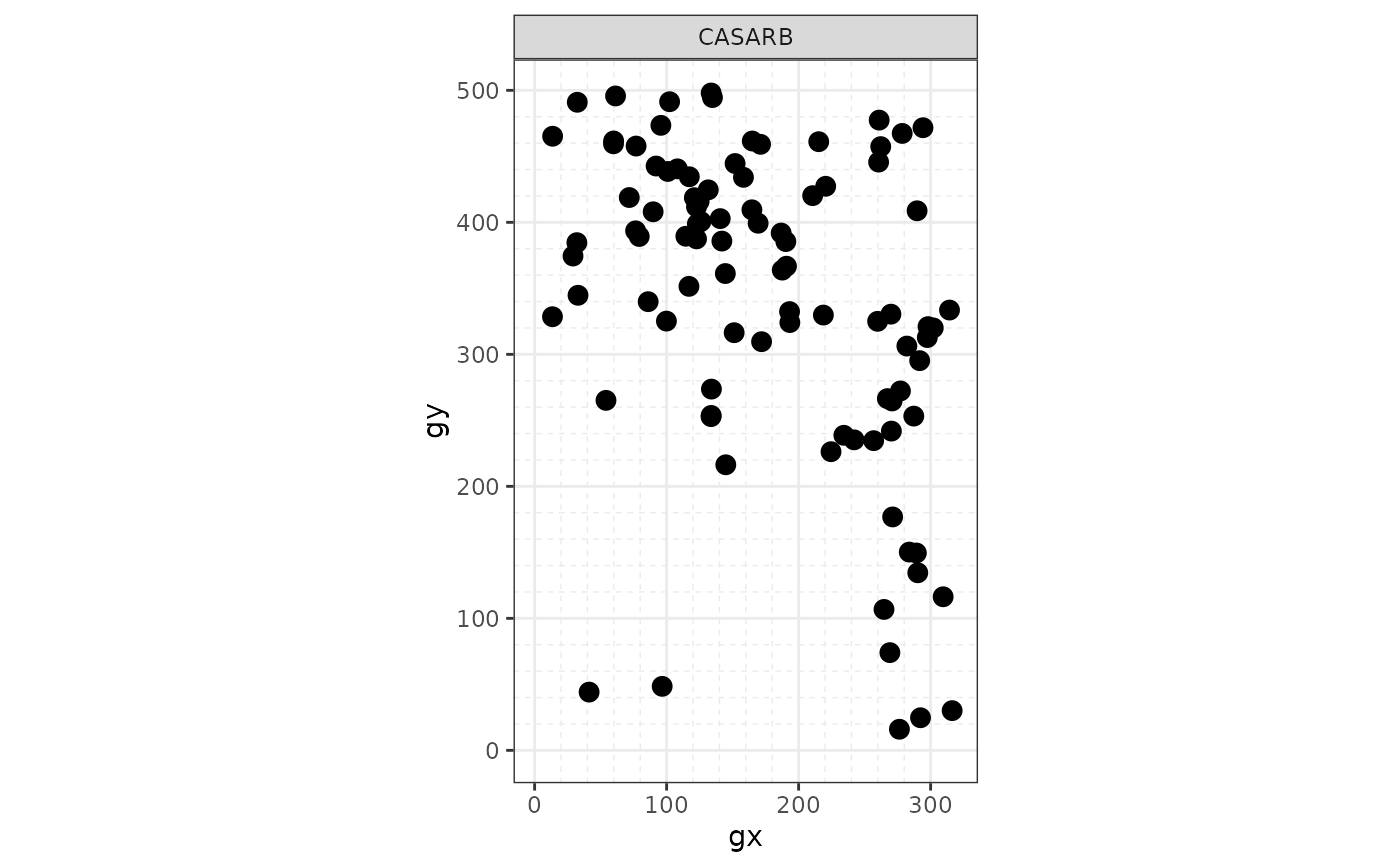#> #>$PREMON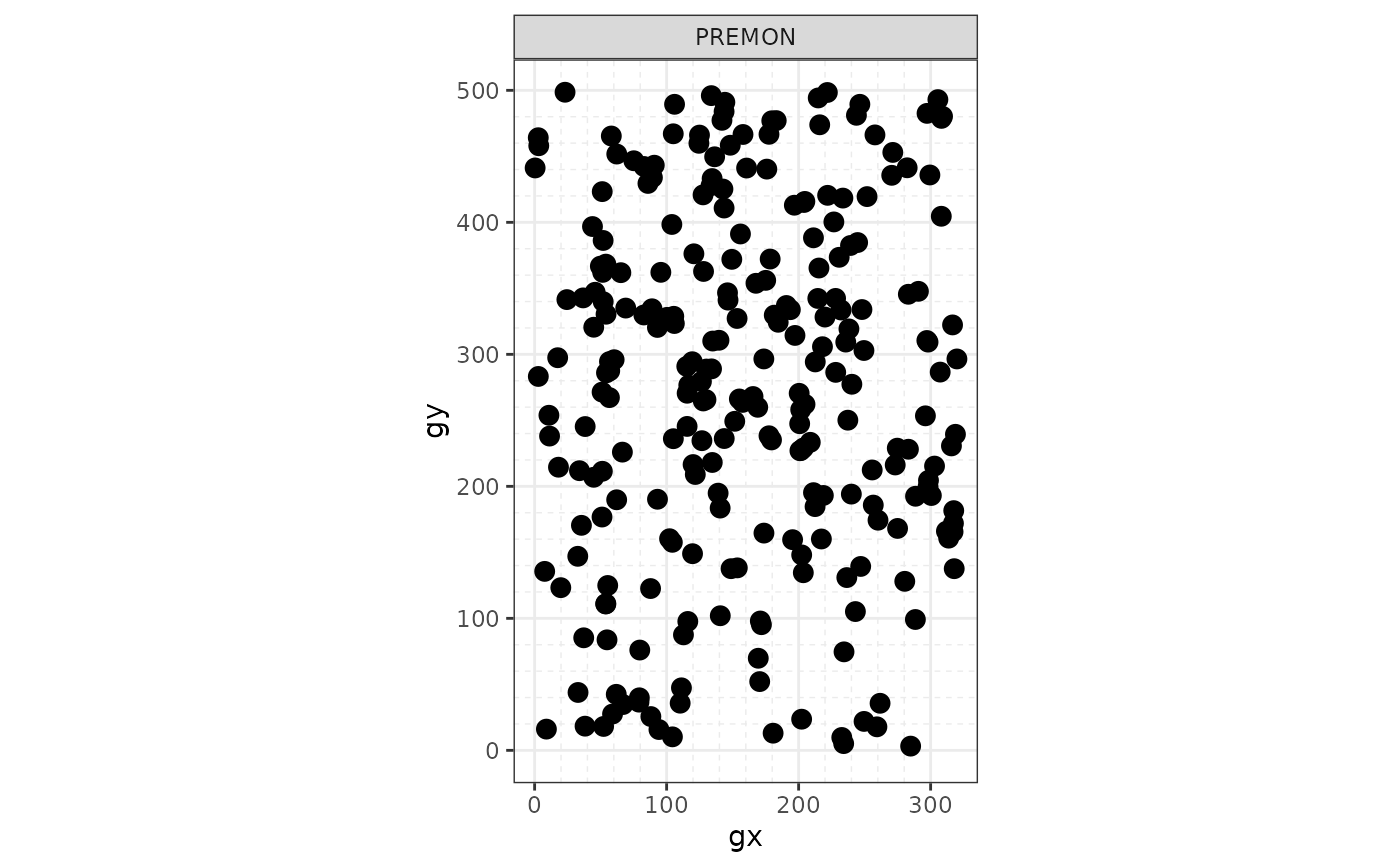#>

# To print all plots in a .pdf see ?pdf()
autoplot_by_species(sp(census))
#> $CASARB#> #>$PREMON#>
#> $SLOBER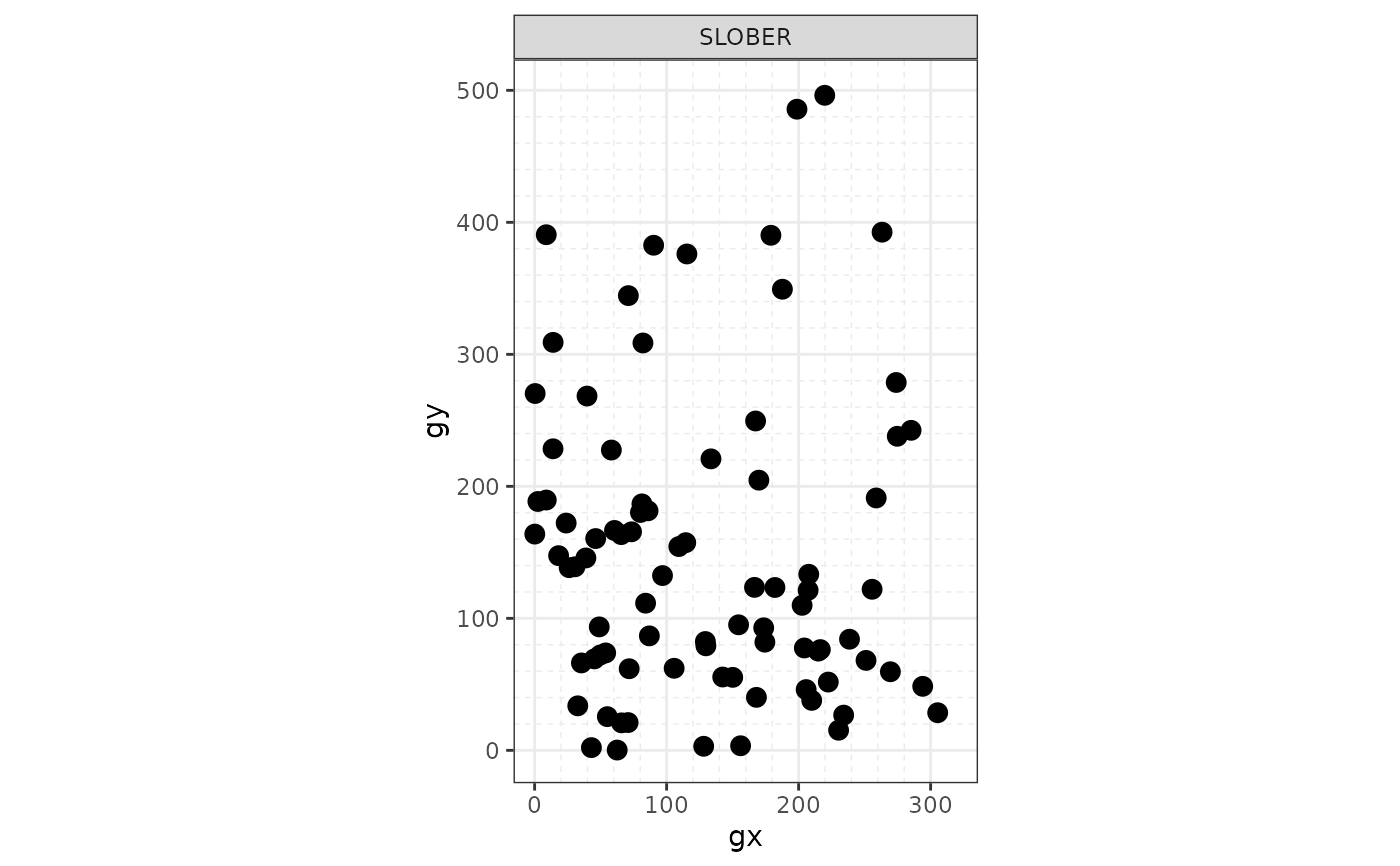#> # Species and elevation (optional) --------------------------------------- # Species and elevation elevation <- fgeo.x::elevation autoplot_by_species(sp_elev(census, elevation)) #>$CASARB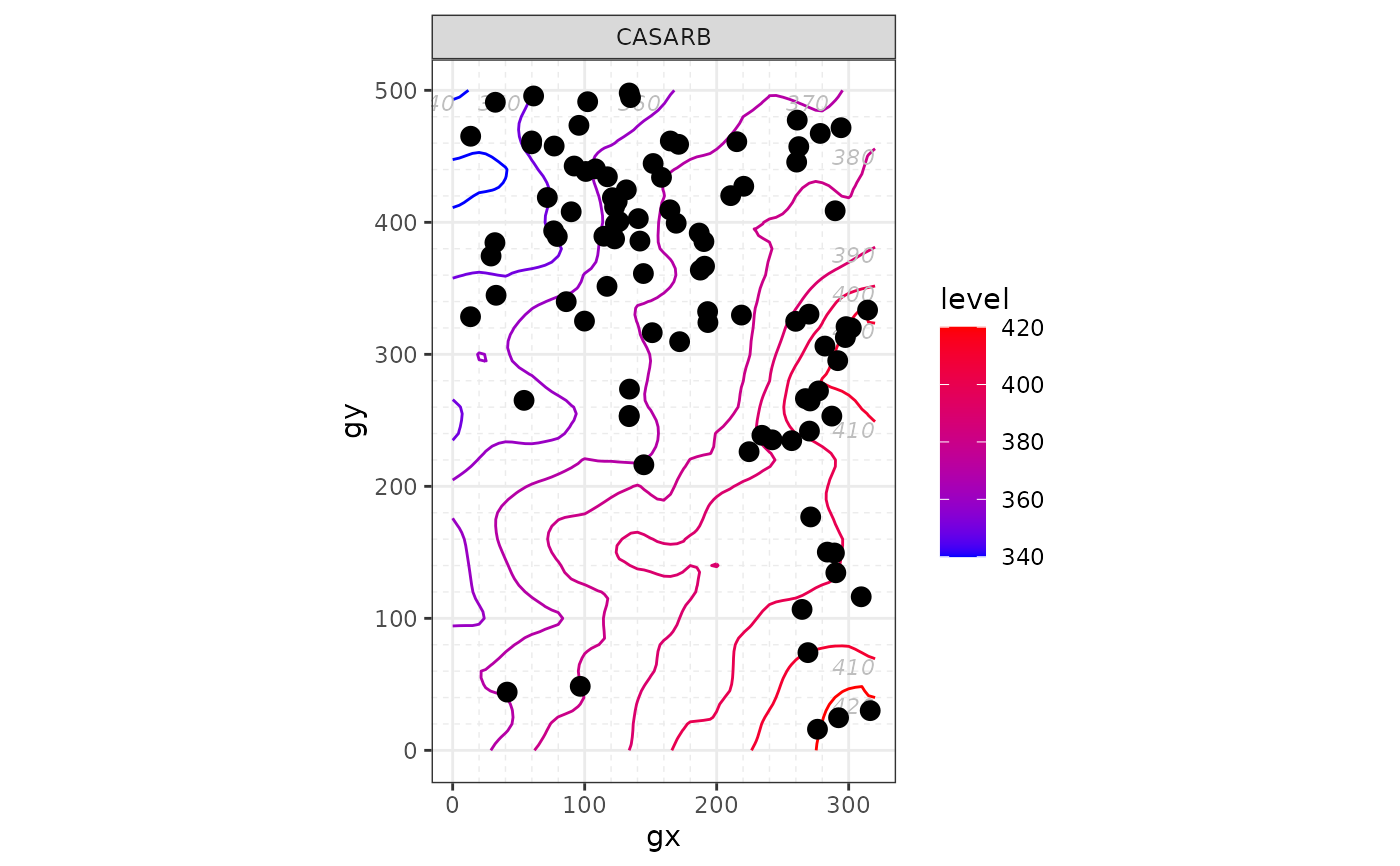#>
#> $PREMON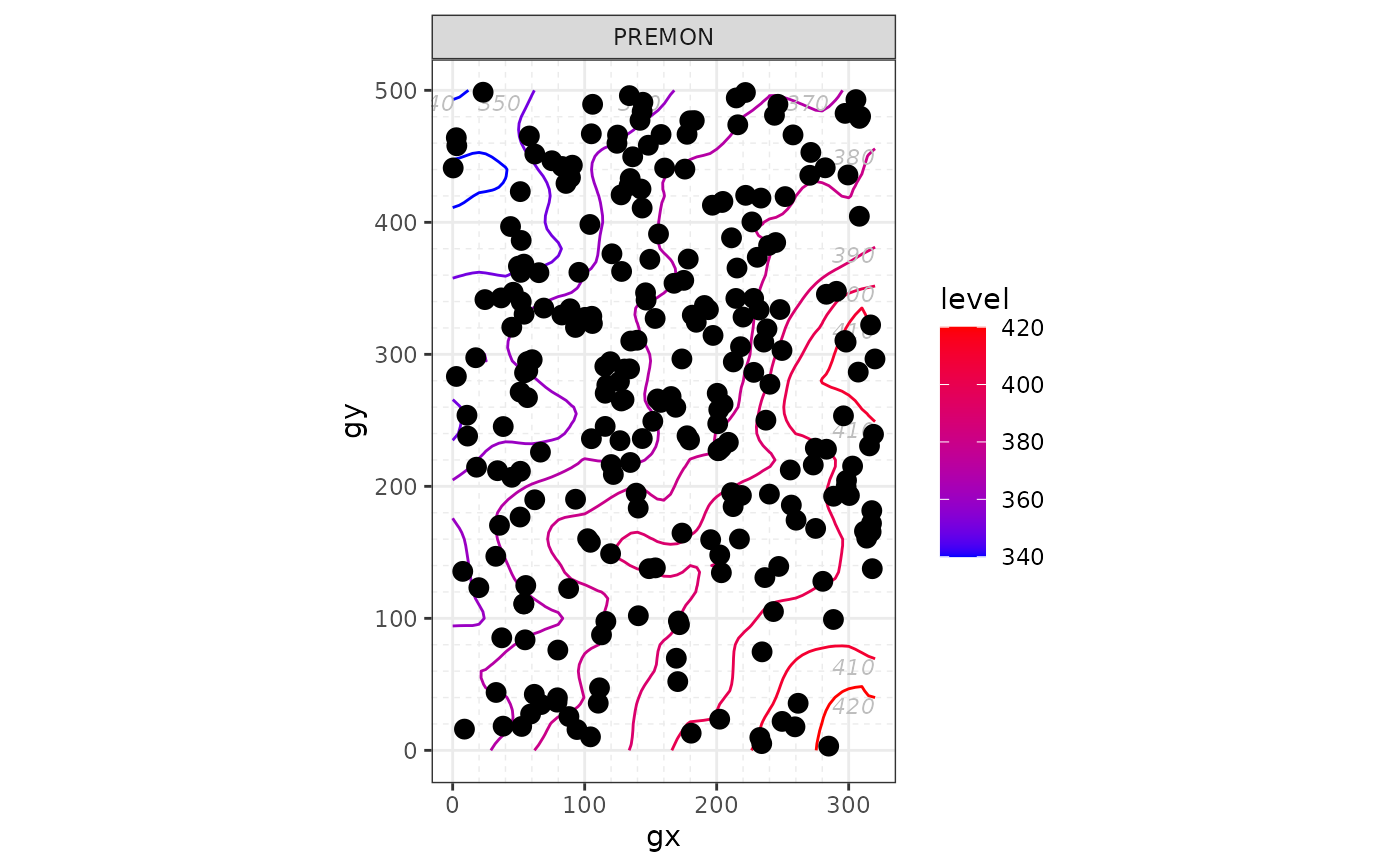#> #>$SLOBER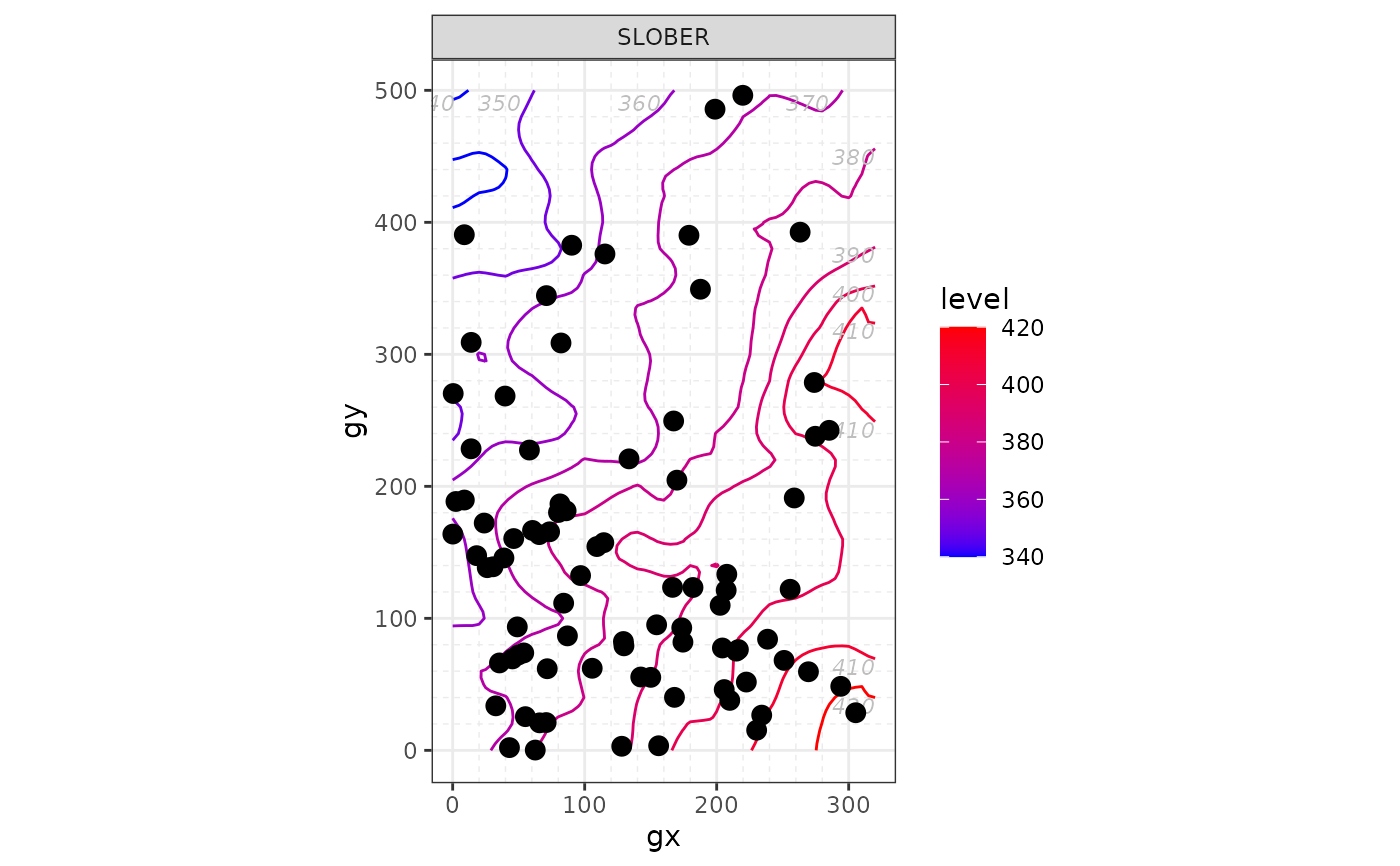#>